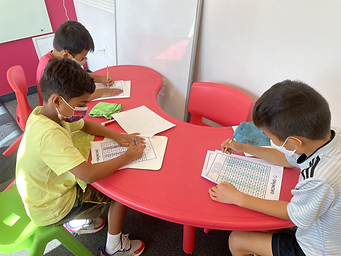## Ms. Caitlyn

### Target 1​

###### Lesson Type:

New

Number Operation

:

Integer Composition

Recognize a whole number is a multiple of each of its factors.

###### 1:

Determine whether a given whole number in the range of 1 to 100 is a multiple of a given one-digit number.

###### 2:

Using a hundred’s chart, identify all the multiples of two through ten.

###### 3:

Recognize patterns in multiples, setting the foundation for developing computational fluency in multiplication.

4th

###### Vocabulary:

Product, Equation, Multiples, Hundreds Chart

Activities:

Students used a hundreds chart and colored in the multiples of 2, 3, 5, and 7.

Students then competed to find the multiples of the remaining numbers (out of 10) without the use of the hundreds chart.

Students were challenged to find multiples up to 200 using the numbers 11 through 25.### Home Exploration

###### Guiding Questions:## Absent Students:

### Target 2

:

4th

###### Vocabulary:

Prime, Composite, Factors

Activities:

Students discussed what makes a number prime and what makes a number composite.

Students factored different composite numbers.### Home Exploration

###### Guiding Questions:### Target 3

:

###### 1:

Understand there are many ways to multiply multi-digit numbers.

###### 2:

Explain how different methods produce the same product (how each method is doing the same thing).

###### 3:

Understand the role of place-value in multiplication strategies.

###### 4:

Use the standard algorithm for solving multi-digit multiplication equations.

4th

###### Vocabulary:

Place Value, Expanded Form, Lattice Method, Box Method, Multiply

Activities:

Students compared and contrased the different ways that they can do multiplication (expanded form, lattice method, box method, etc.).

Students spun a wheel and rolled dice to generate numbers to create multiplication problems to solve.### Home Exploration